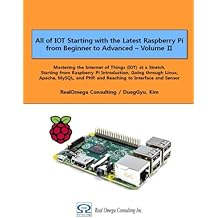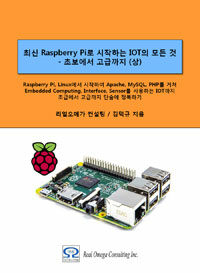# Raspberry Pi_Eng_10.5.1 “expr” Command – Arithmetic Operation

#### Published Book on AmazonAll of IOT Starting with the Latest Raspberry Pi from Beginner to Advanced – Volume 1All of IOT Starting with the Latest Raspberry Pi from Beginner to Advanced – Volume 2

#### 출판된 한글판 도서최신 라즈베리파이(Raspberry Pi)로 시작하는 사물인터넷(IOT)의 모든 것 – 초보에서 고급까지 (상)최신 라즈베리파이(Raspberry Pi)로 시작하는 사물인터넷(IOT)의 모든 것 – 초보에서 고급까지 (하)

## 10.5Commands Related to Operation

### 10.5.1"expr" Command – Arithmetic Operation

You can use the "expr" command to perform arithmetic operations.

[Command Format]

 expr [OPTION] / [EXPRESSION]

[Command Overview]

This performs arithmetic operation on the specified operation expression.

User privilege          -- Normal user.

[Detail Description]

This command basically requires spaces for operand and operator.

Use ` ` (backtab) to store the values computed using this command elsewhere.

Note that you must use escape () when you use "*".

[Main Option]

 --help display this help and exit --version output version information and exit

[주요 expression]

 ARG1 | ARG2 ARG1 if it is neither null nor 0, otherwise ARG2 ARG1 & ARG2 ARG1 if neither argument is null or 0, otherwise 0 ARG1 < ARG2 if ARG1 is less than ARG2, then 1, otherwise 0 ARG1 <= ARG2 if ARG1 is less than or equal to ARG2, then 1, otherwise 0 ARG1 = ARG2 if ARG1 is equal to ARG2, then 1, otherwise 0 ARG1 != ARG2 if ARG1 is unequal to ARG2, then 1, otherwise 0 ARG1 >= ARG2 if ARG1 is greater than or equal to ARG2, then 1, otherwise 0 ARG1 > ARG2 if ARG1 is greater than ARG2, then 1, otherwise 0 ARG1 + ARG2 arithmetic sum of ARG1 and ARG2 ARG1 - ARG2 arithmetic difference of ARG1 and ARG2 ARG1 * ARG2 arithmetic product of ARG1 and ARG2 ARG1 / ARG2 arithmetic quotient of ARG1 divided by ARG2 ARG1 % ARG2 arithmetic remainder of ARG1 divided by ARG2

[Used Example]

The followings are some of the examples that use this command

 pi@raspberrypi ~ \$ echo `expr 5 + 4' 9 pi@raspberrypi ~ \$ echo `expr 11 – 6' 5 pi@raspberrypi3:~ \$ echo `expr 10 "|" 75` 10 pi@raspberrypi3:~ \$ echo `expr 0 "|" 75` 75 pi@raspberrypi3:~ \$ echo `expr 10 ">" 75` 0 pi@raspberrypi3:~ \$ echo `expr 10 ">" 7` 1 pi@raspberrypi3:~ \$ echo `expr 10 "<" 7` 0 pi@raspberrypi3:~ \$ echo `expr 10 "<" 75` 1